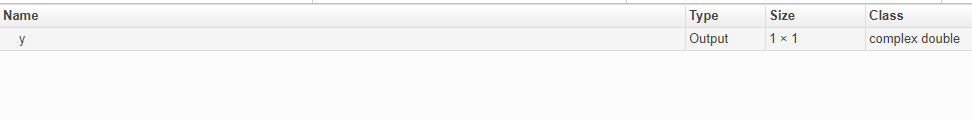Documentation

## Code Generation for Complex Data

### Restrictions When Defining Complex Variables

For code generation, you must set the complexity of variables at the time of assignment. Assign a complex constant to the variable or use the `complex` function. For example:

```x = 5 + 6i; % x is a complex number by assignment. y = complex(5,6); % y is the complex number 5 + 6i. ```

After assignment, you cannot change the complexity of a variable. Code generation for the following function fails because ```x(k) = 3 + 4i``` changes the complexity of `x`.

```function x = test1( ) x = zeros(3,3); % x is real for k = 1:numel(x) x(k) = 3 + 4i; end end```

To resolve this issue, assign a complex constant to `x`.

```function x = test1( ) x = zeros(3,3)+ 0i; %x is complex for k = 1:numel(x) x(k) = 3 + 4i; end end```

### Code Generation for Complex Data with Zero-Valued Imaginary Parts

For code generation, complex data that has all zero-valued imaginary parts remains complex. This data does not become real. This behavior has the following implications:

• In some cases, results from functions that sort complex data by absolute value can differ from the MATLAB® results. See Functions That Sort Complex Values by Absolute Value.

• For functions that require that complex inputs are sorted by absolute value, complex inputs with zero-valued imaginary parts must be sorted by absolute value. These functions include `ismember`, `union`, `intersect`, `setdiff`, and `setxor`.

#### Functions That Sort Complex Values by Absolute Value

Functions that sort complex values by absolute value include `sort`, `issorted`, `sortrows`, `median`, `min`, and `max`. These functions sort complex numbers by absolute value even when the imaginary parts are zero. In general, sorting the absolute values produces a different result than sorting the real parts. Therefore, when inputs to these functions are complex with zero-valued imaginary parts in generated code, but real in MATLAB, the generated code can produce different results than MATLAB. In the following examples, the input to `sort` is real in MATLAB, but complex with zero-valued imaginary parts in the generated code:

#### Inputs and Outputs for MEX Functions Generated for Complex Arguments

For MEX functions created by MATLAB Coder™ :

• Suppose that you generate the MEX function for complex inputs. If you call the MEX function with real inputs, the MEX function transforms the real inputs to complex values with zero-valued imaginary parts.

• If the MEX function returns complex values that have all zero-valued imaginary parts, the MEX function returns the values to the MATLAB workspace as real values. For example, consider this function:

```function y = foo() y = 1 + 0i; % y is complex with imaginary part equal to zero end ```

If you generate a MEX function for `foo` and view the code generation report, you see that `y` is complex.

`codegen foo -report`If you run the MEX function, you see that in the MATLAB workspace, the result of `foo_mex` is the real value `1`.

`z = foo_mex `
```ans = 1```

### Results of Expressions That Have Complex Operands

In general, expressions that contain one or more complex operands produce a complex result in generated code, even if the value of the result is zero. Consider the following line of code:

```z = x + y; ```

Suppose that at run time, `x` has the value ```2 + 3i``` and `y` has the value ```2 - 3i```. In MATLAB, this code produces the real result `z = 4`. During code generation, the types for `x` and `y` are known, but their values are not known. Because either or both operands in this expression are complex, `z` is defined as a complex variable requiring storage for a real and an imaginary part. `z` equals the complex result `4 + 0i` in generated code, not `4`, as in MATLAB code.

Exceptions to this behavior are:

• When the imaginary parts of complex results are zero, MEX functions return the results to the MATLAB workspace as real values. See Inputs and Outputs for MEX Functions Generated for Complex Arguments.

• When the imaginary part of the argument is zero, complex arguments to extrinsic functions are real.

```function y = foo() coder.extrinsic('sqrt') x = 1 + 0i; % x is complex y = sqrt(x); % x is real, y is real end ```
• Functions that take complex arguments but produce real results return real values.

```y = real(x); % y is the real part of the complex number x. y = imag(x); % y is the real-valued imaginary part of x. y = isreal(x); % y is false (0) for a complex number x. ```
• Functions that take real arguments but produce complex results return complex values.

```z = complex(x,y); % z is a complex number for a real x and y. ```

### Results of Complex Multiplication with Nonfinite Values

When an operand of a complex multiplication contains a nonfinite value, the generated code might produce a different result than the result that MATLAB produces. The difference is due to the way that code generation defines complex multiplication. For code generation:

• Multiplication of a complex value by a complex value (a + bi) (c + di) is defined as (ac - bd) + (ad + bc)i. The complete calculation is performed, even when a real or an imaginary part is zero.

• Multiplication of a real value by a complex value c(a + bi) is defined as ca + cbi .

##### Support평가판 신청